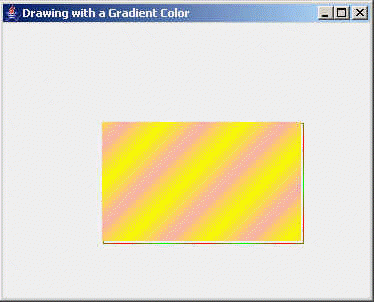Tutorials

# Drawing with Gradient Color in Java

In this section, you will learn how to draw the colorful gradient shapes.

In this section, you will learn how to draw the colorful gradient shapes.

# Drawing with Gradient Color in Java

In this section, you will learn how to draw the colorful gradient shapes. First of all you will know about the gradient color. The gradient color is combination of more than one colors to design graphics. Object with the gradient color looks like a 3-D component. The object colored with gradient color makes it more attractive. The screen shot is given below:Here you will see the gradient colors have been used in the Rectangle with the help of  this program. Program draws a rectangle using Graphics2D and GradientPaint()

Code Description:

The Gradient color uses the following methods:

Graphics2D:
This is the class which extends from the Graphics class i.e. used to control the geometry according to the coordinate. It is also used to manage texts.

This is a constructor of Gradient class. It is used to create a gradient color with the help of getGradient() method.

This is a constructor of Gradient class. It used to fill the gradient color in the shapes.

GradientPaint(startX, startY, startColor, endX, endY, endColor, true)

startX - It represents the X-coordinate of the starting point.
startY - It represents the Y-coordinate of thestarting point.
startColor - It is used to set the starting color of the shape.
endX
- It represents the X-coordinate of the ending point.
endY
- It represents the Y- coordinate of the ending point.
endColor - It is used to set the ending color of the shape.
true
- It is a boolean value mentioned for the cyclic presentation of color in the shape mean the starting color and the ending color are repeated up to the size of the shape.

Here is the code of program:

 ``` import javax.swing.*; import java.awt.*; public class GradientColor{   public static void main(String[] args) {   GradientColor gd = new GradientColor();   }      public GradientColor(){   JFrame frame = new JFrame("Drawing with a Gradient Color");   frame.setDefaultCloseOperation(JFrame.EXIT_ON_CLOSE);   frame.getContentPane().add(new MyComponent());   frame.setSize(400,400);   frame.setVisible(true);   }   public class MyComponent extends JComponent  {   public void paint(Graphics g){   Graphics2D g2d = (Graphics2D)g;   Color s1 = Color.red;   Color e = Color.green;   GradientPaint gradient = new GradientPaint(10,10,s1,30,30,e,true);   g2d.setPaint(gradient);   g2d.drawRect(100,100,200,120);   Color s2 = Color.yellow;   Color e1 = Color.pink;   GradientPaint gradient1 = new GradientPaint(10,10,s2,30,30,e1,true);   g2d.setPaint(gradient1);   g2d.fillRect(99,99,199,119);   }   } }```

```Download this example.```